# 平面剪切来流作用下串列布置三圆柱流致运动特性研究1)

*湘潭大学 土木工程与力学学院，湖南湘潭 411105
†岩土力学与工程安全湖南省重点实验室，湖南湘潭 411105

# STUDY OF FLOW-INDUCED MOTION CHARACTERISTICS OF THREE TANDEM CIRCULAR CYLINDERS IN PLANAR SHEAR FLOW1)

Tu Jiahuang*2), Tan Xiaoling*, Deng Xuhui*, Guo Xiaogang*, Liang Jingqun*, Zhang Ping*

* College of Civil Engineering and Mechanics， Xiangtan University， Xiangtan 411105， Hunan， China
† Hunan Key Laboratory of Geomechanics and Engineering Safety， Xiangtan 411105， Hunan， China

Abstract

Flow-induced motions of three elastically mounted circular cylinders subjected to the planar shear flow in tandem arrangement at $Re =100$ were numerically investigated by using semi-implicit characteristics-based split (CBS) finite element algorithm. Firstly, the results are compared with the existing data in the literature to verify the accurateness of the method. Then, the influence of some key parameters, such as shear ratio ($k$), natural frequency ratio ($r$) and reduced velocity ($U_{\rm r}$) on the characteristics of flow-induced dynamic responses and flow field of three circular cylinders with tandem arrangement was analyzed. The numerical results show that the shear ratio, natural frequency ratio and reduced velocity play an important role in the amplitude and trajectory of motion. The change of maximum amplitude of the upstream cylinder is similar to that of an isolated cylinder case with the increasing of $k$. The maximum amplitudes of the midstream and downstream cylinders increase, and the dual resonance is observed. Meanwhile, the increasing of $k$ causes the extension of the resonance region. With the increasing of $r$, the lock-in region of the upstream cylinder reduces in the in-line direction. However, for the midstream and downstream ones, the dual-lock-in region broadens. On the other hand, the prominent $X-Y$ trajectories of three circular cylinder resemble figure-eight shape and irregular one in the uniform flow. With the change of $k$, the motion orbit transforms from figure-eight to the raindrop shape in the lock-in region. In the larger shear ratio and higher natural frequency ratio case, the dual-raindrop is observed in the $X-Y$ vibrating trajectory of the midstream cylinder. Finally, the flow-induced motion mechanism of three tandem circular cylinders exposed to planar shear flow is revealed according to the analysis of the characteristic of the flow field.

Keywords： planar shear flow ; three tandem circular cylinders ; flow induced motion ; dynamic response ; flow characteristics

0

Tu Jiahuang, Tan Xiaoling, Deng Xuhui, Guo Xiaogang, Liang Jingqun, Zhang Ping. STUDY OF FLOW-INDUCED MOTION CHARACTERISTICS OF THREE TANDEM CIRCULAR CYLINDERS IN PLANAR SHEAR FLOW1)[J]. Chinese Journal of Theoretical and Applied Mechanics, 2019, 51(3): 787-802 https://doi.org/10.6052/0459-1879-18-348

## 1 数值方法

### 1.1 流体控制方程

$\dfrac{\partial {\pmb u}_i }{\partial x_i } = {\bf 0} (1)$

$\dfrac{\partial {\pmb u}_i }{\partial t} + {\pmb c}_j \dfrac{\partial {\pmb u}_i }{\partial x_j } = - \dfrac{\partial {\pmb p}}{\partial x_i } + \dfrac{1}{Re}\dfrac{\partial ^2{\pmb u}_i }{\partial x_j \partial x_j } (2)$

### 1.2 四步半隐式CBS稳定化流体有限元

$\dfrac{{\pmb u}_i^1 - {\pmb u}_i^{(n)} }{\Delta t} = - {\pmb u}_j^{(n)} \dfrac{\partial {\pmb u}_i^{(n)} }{\partial X_j } + \dfrac{\Delta t}{2}{\pmb u}_m^{(n)} \dfrac{\partial }{\partial X_m }\left( {{\pmb u}_j^{(n)} \dfrac{\partial {\pmb u}_i^{(n)} }{\partial X_j }} \right) (3)$

$\dfrac{\partial ^2{\pmb p}^{\left( {n + 1} \right)}}{\partial X_i^2 } = \dfrac{1}{\Delta t}\dfrac{\partial {\pmb u}_i^1 }{\partial X_i } (4)$

$\dfrac{{\pmb u}_i^2 - {\pmb u}_i^1 }{\Delta t} = - \dfrac{\partial {\pmb p}^{n + 1}}{\partial X_i } (5)$

$\dfrac{{\pmb u}_i^{\left( {n + 1} \right)} - {\pmb u}_i^2 }{\Delta t} = \dfrac{1}{Re}\dfrac{\partial ^2{\pmb u}_i^{\left( {n + 1} \right)} }{\partial X_j \partial X_j } (6)$

$${\pmb u}_i = \sum_{I = 1}^3 {N_I } {\pmb u}_{iI} \,, \ \ {\pmb p} = \sum_{I = 1}^3 {N_I } {\pmb p}_I (7)$$

${\pmb A}_{IJ} \dfrac{{\pmb u}_{iJ}^1 - {\pmb u}_{iJ}^{(n)} }{\Delta t} = - \left( {{\pmb B}_{IJ}^{(n)} + {\pmb C}_{S,IJ}^{(n)} } \right) {\pmb u}_{iJ}^{(n)} (8)$

${\pmb D}_{IJ} {\pmb P}_J^{\left( {n + 1} \right)} = - \dfrac{1}{\Delta t}{\pmb E}_{iIJ} {\pmb u}_{iJ}^1 (9)$

${\pmb A}_{IJ} \dfrac{{\pmb u}_{iJ}^2 - {\pmb u}_{iJ}^1 }{\Delta t} = - {\pmb E}_{iIJ} {\pmb P}_J^{\left( {n + 1} \right)} (10)$

$\left( {{\pmb A}_{IJ} + \dfrac{\Delta t}{Re}{\pmb D}_{IJ} } \right) {\pmb u}_{iJ}^{\left( {n + 1} \right)} = {\pmb A}_{IJ} {\pmb u}_{iJ}^2 (11)$

$\left.\begin{matrix} {\pmb A}_{IJ} = \int_\varOmega {N_I } N_J \varOmega \ \ {\pmb B}_{IJ}^{(n)} = \int_\varOmega {N_I } {\pmb u}_j^{(n)} \dfrac{\partial N_J }{\partial X_j } \varOmega \\ {\pmb C}_{S,IJ}^{(n)} = \dfrac{\Delta t}{2}\int_\varOmega {\dfrac{\partial N_I }{\partial X_m }} {\pmb u}_m^{(n)} {\pmb u}_j^{(n)} \dfrac{\partial N_J }{\partial X_j }\varOmega \\ {\pmb D}_{IJ} = \int_\varOmega {\dfrac{\partial N_I }{\partial X_i }} \dfrac{\partial N_J }{\partial X_i }\varOmega \,, \ \ {\pmb E}_{iIJ} = \int_\varOmega {N_I \dfrac{\partial N_J }{\partial X_i }} \varOmega \\\end{matrix} \right\}$

### 1.3 固体控制方程

$\ddot {\pmb X} + \dfrac{4\pi \xi }{{\pmb U}_{{\rm r},x} }\dot{\pmb X} + \dfrac{4\pi ^2}{{\pmb U}_{{\rm r},x}^2 }X = \dfrac{{\pmb C}_{\rm D} }{2m_{\rm r} } (13)$

$\ddot {\pmb Y} + \dfrac{4\pi \xi }{{\pmb U}_{{\rm r},y} }\dot{\pmb Y} + \dfrac{4\pi ^2}{{\pmb U}_{{\rm r},y}^2 }Y = \dfrac{{\pmb C}_{\rm L} }{2m_{\rm r} } (14)$

### 1.4 网格更新

$$\dfrac{\partial ^2\left[ {\left( {1 + \tau } \right) {\pmb S}_i } \right]}{\partial x_j \partial x_j } = {\bf 0}\,, \ \ {\pmb S}_i = {\pmb g}_i \left| {_{\varGamma _{\rm m }} } \right.\,, \ \ {\pmb S}_i = {\bf 0}\left| {_{\varGamma _{\rm f }} } \right. (15)$$

### 1.5 流固耦合计算步骤

(1) 求解流体控制方程：运用基于四步半隐式CBS稳定化有限元方法求解流体控制方程(1)和(2),获得$t^{(n + 1)}$时刻流场速度与压力,从而得到流体作用于结构上的流体力.

(2) 求解固体控制方程：将流体力($C_{\rm D}$, $C_{\rm L}$)施加到结构,以Newmark-$\beta$时间积分方法求解结构运动控制方程 (13)和(14),得到$t^{(n + 1)}$时刻结构的动力响应.

(3) 网格更新：采用标准Galerkin有限元方法求解Laplace方程,获得网格节点位移及网格速度,并更新网格坐标.

(4) 返回第一步开始计算$t^{(n + 2)}$时刻,并循环至系统达到稳定为止.

### 1.6 算例验证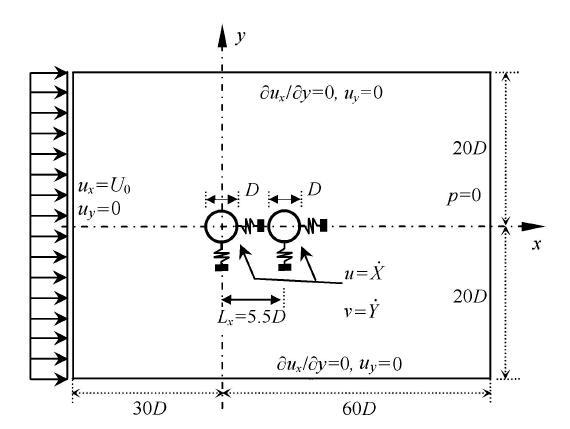Fig. 1   Model schematic of validation study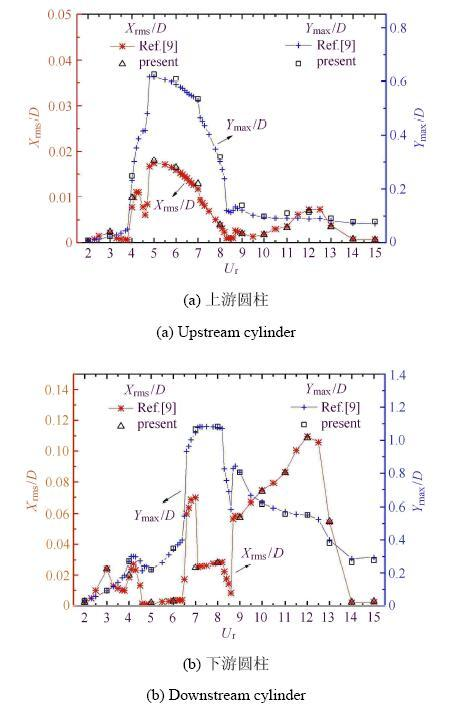Fig. 2   The comparisons of the variation of vibration amplitudes with $U_{\rm r}$

## 2 问题描述

### 2.1 计算模型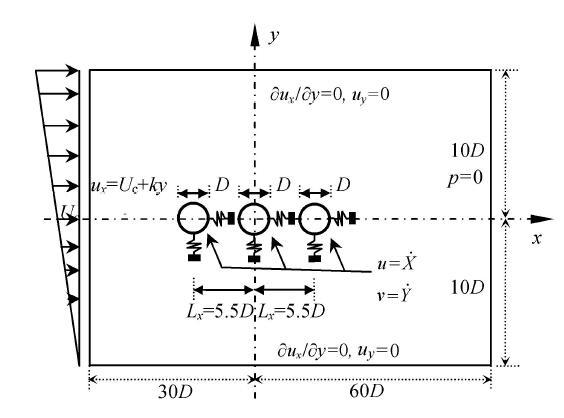Fig. 3   The computational model and boundary conditions

### 2.2 网格划分

Table 1   Grid independence test: the results for the three circular cylinders in the tandem arrangement at ${Re}=100$, $k=0$, $r=1.0$, $U_{\rm r} =5.5$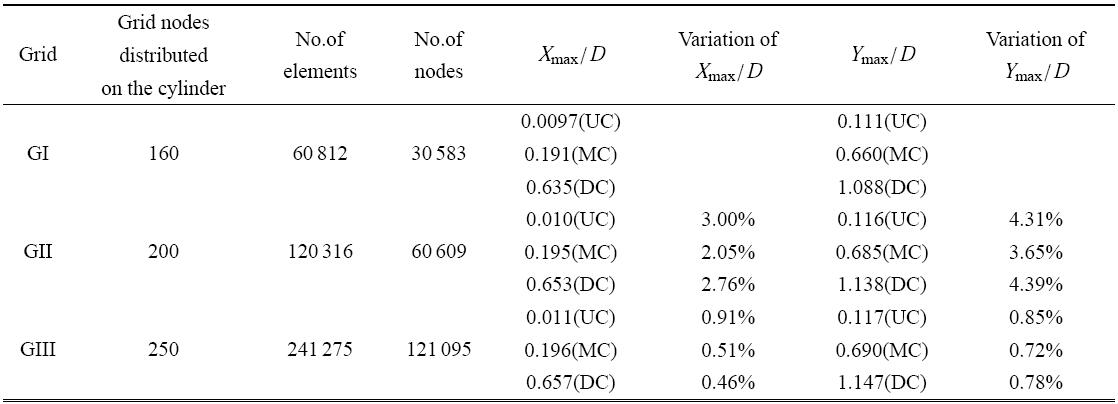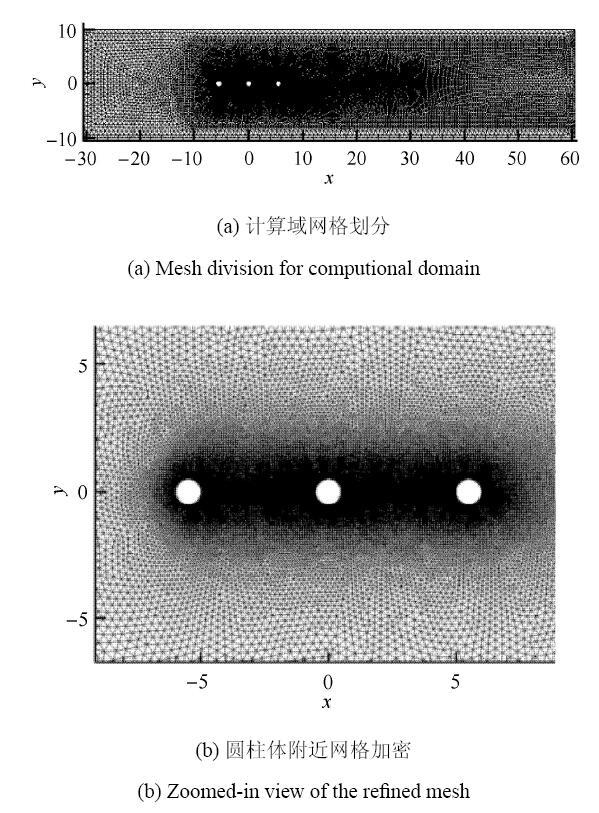Fig. 4   Schematic of the computational mesh

## 3 计算结果与分析

### 3.1 振幅特性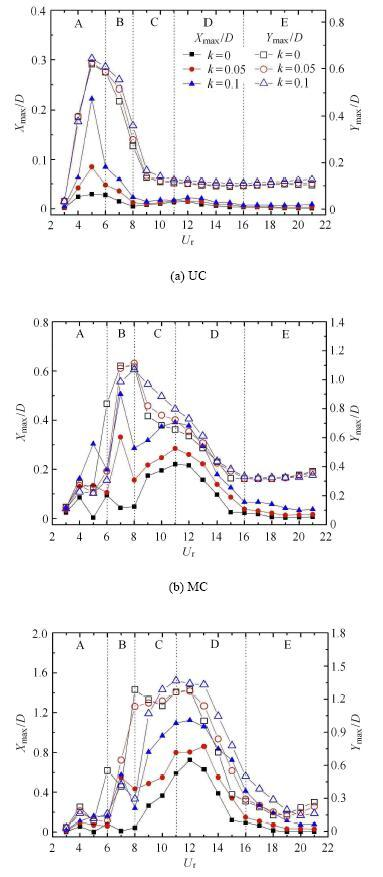Fig. 5   The variation of the maximum vibrating amplitudes with reduced velocity under different shear ratios ($r =1.0$)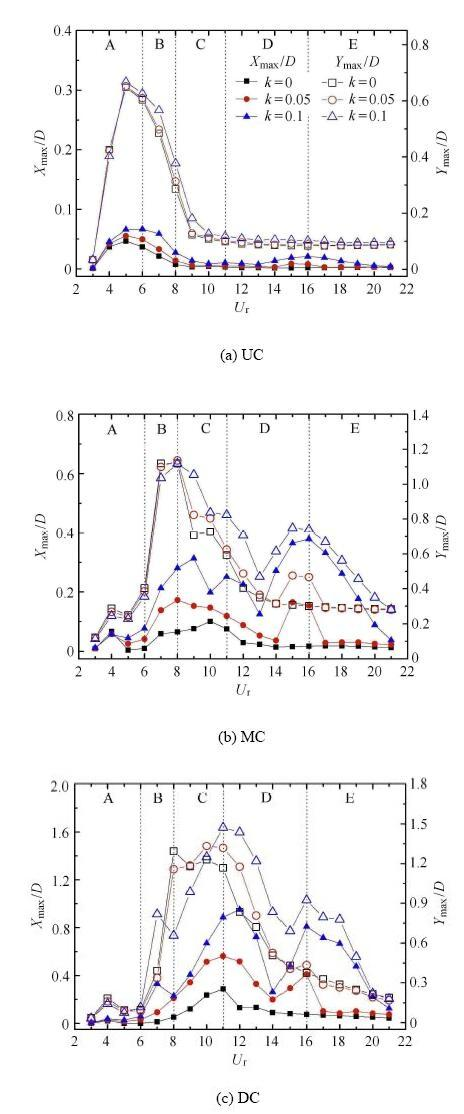Fig. 6   The variation of the maximum vibrating amplitudes with reduced velocity under different shear ratios ($r =1.5$)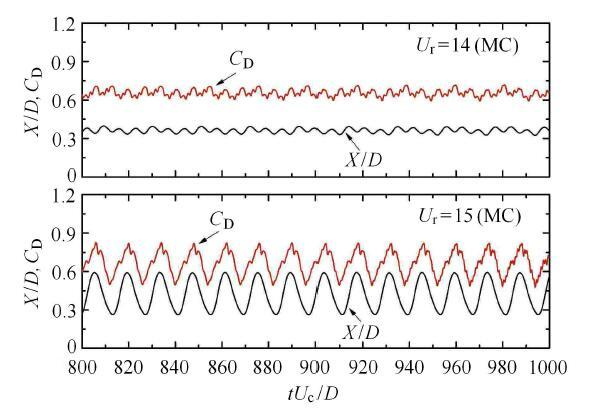Fig. 7   Time history of streamwise displacements ($X/D)$ and the drag coefficients ($C_{\rm D})$ at different reduced velocities ($k =0.05$, $r =1.5$)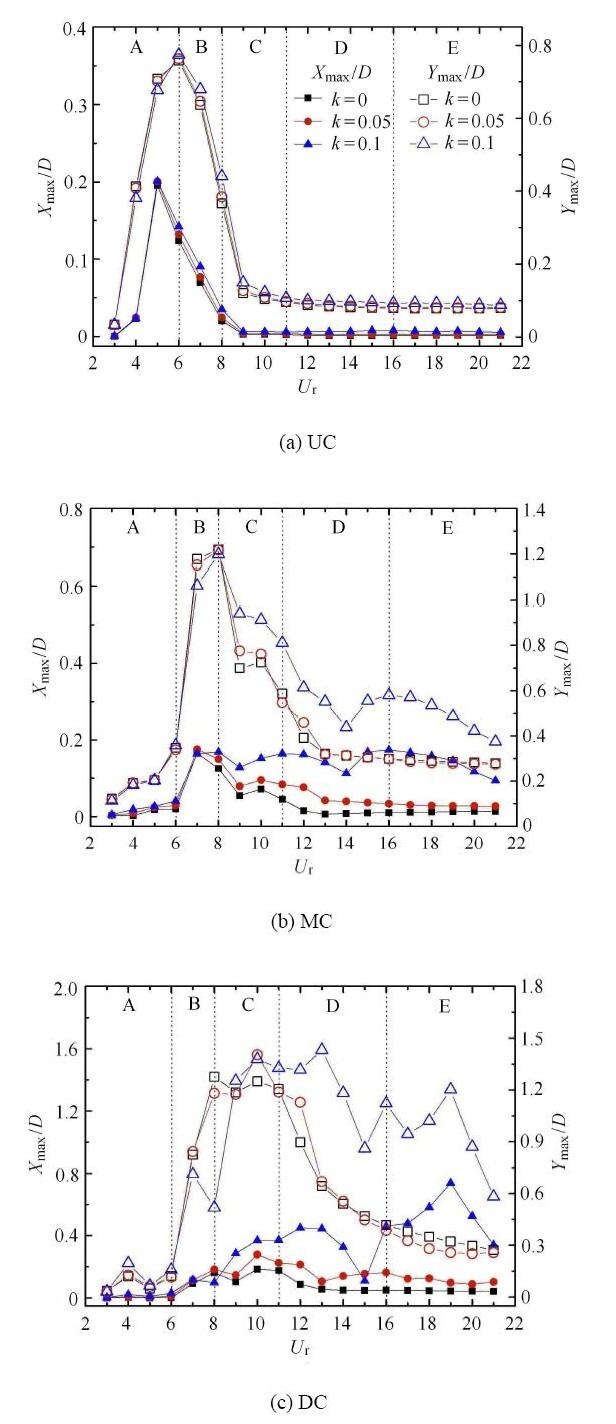Fig. 8   The variation of the maximum vibratin amplitudes with reduced velocity under different shear ratios ($r =2.0$)

### 3.2 频率特性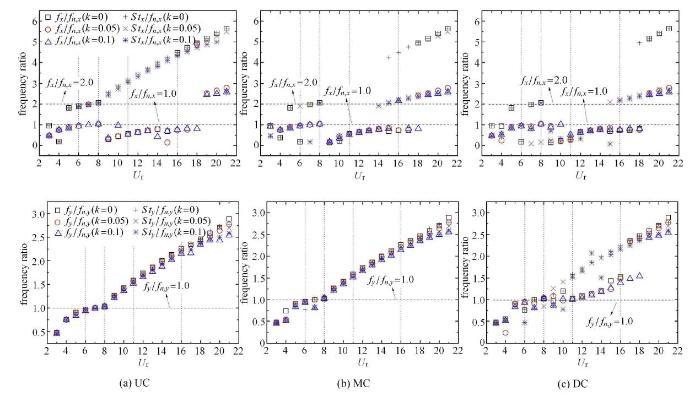Fig. 9   The variation of frequency ratios with reduced velocities under different shear ratios ($r =1.0$)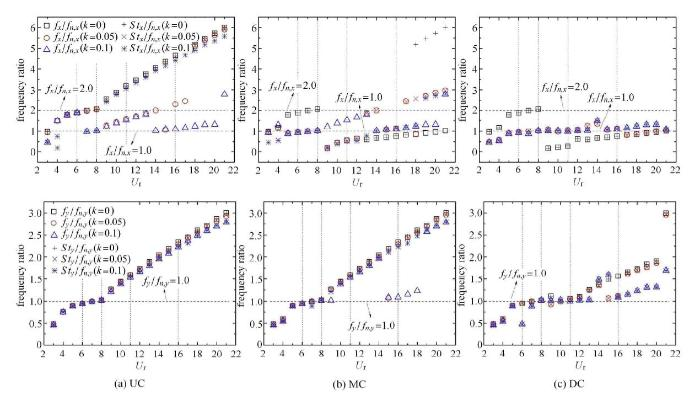Fig. 10   The variation of frequency ratios with reduced velocitiesunder different shear ratios ($r =1.5$)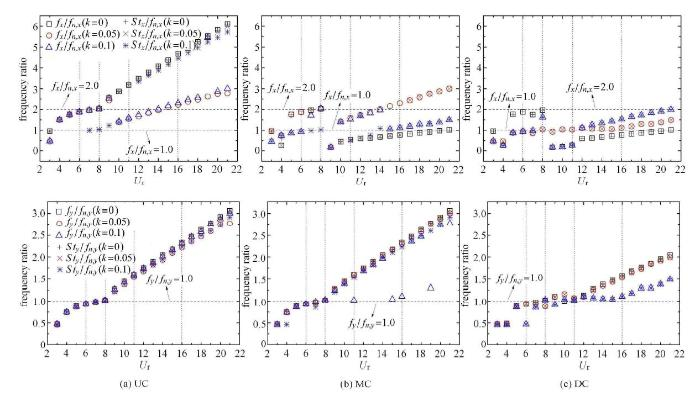Fig. 11   The variation of frequency ratios with reduced velocities under different shear ratios ($r =2.0$)

### 3.3 运动轨迹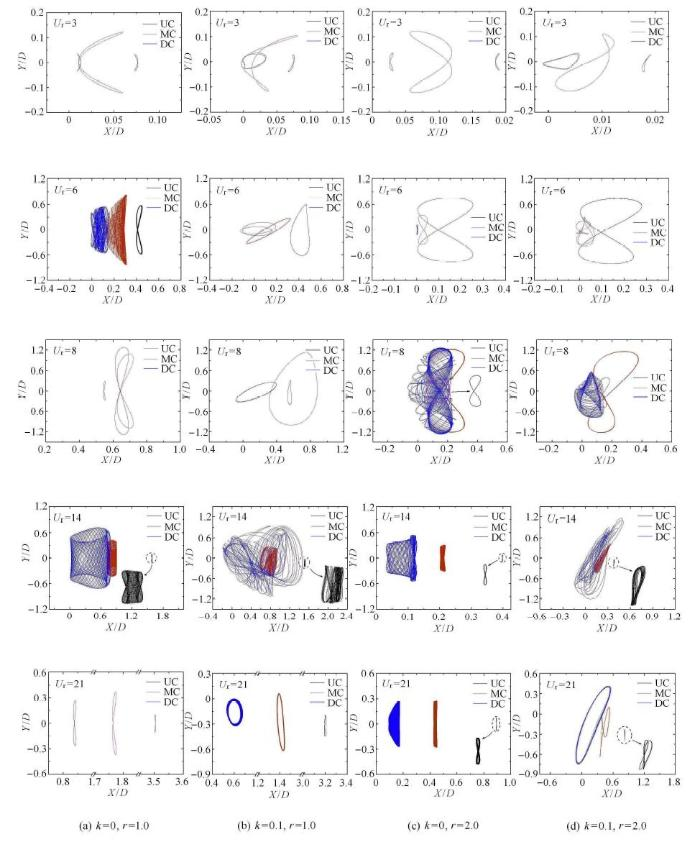Fig. 12   The variation of $X$-$Y$ trajectories with reduced velocities at different cases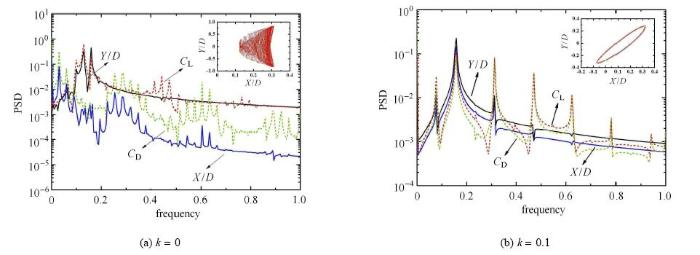Fig. 13   Power spectral density (PSD) of the flow force coefficients($C_{\rm D}$, $C_{\rm L})$ and displacements ($X/D$, $Y/D)$ of midstream cylinder under different shear ratios ($r =1.0$, $U_{\rm r} =6$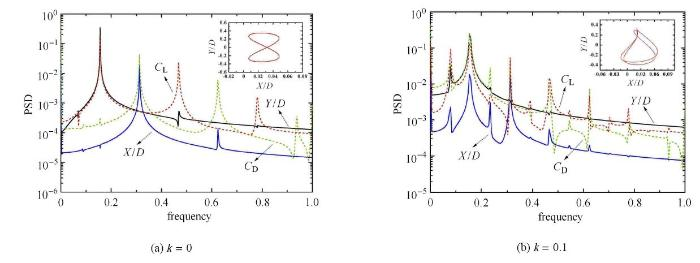Fig. 14   Power spectral density (PSD) of the flow force coefficients ($C_{\rm D}$, $C_{\rm L})$ and displacements ($X/D$, $Y/D)$ of midstream cylinder under different shear ratios ($r =2.0$, $U_{\rm r} =6$)

## 4 涡动力机理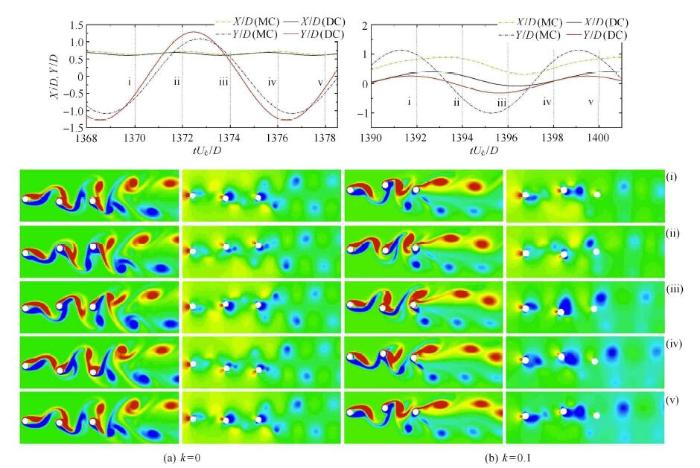Fig. 15   Time-history curve of displacement of midstream and downstream cylinders under different shear ratio cases and vorticity contours and instantaneous pressure contours at different times ($r =1.0$, $U_{\rm r} =8$)

## 5 结 论

The authors have declared that no competing interests exist.

## 参考文献 原文顺序 文献年度倒序 文中引用次数倒序 被引期刊影响因子

(Luo Zhumei, Zhang Lixiang.Force characteristics and hydrokinetic energy harvesting for VIV of four coupling-linked cylinders. Journal of vibration and shock, 2015, 34(17): 25-29 (in Chinese)) 涡激振动是一种常见的流固耦合现象，结构物振幅较大时，可利用涡激振动从海洋流中或河流中获取能量。论文通过流固双向耦合数值方法，模拟均匀来流下，弹性支撑刚性连接的耦合四圆柱在不同横向和流向间距比下的横向涡激振动。分析横向和流向间距比对该耦合结构涡激振动时的压力和升力特性及获取能量和能量密度的影响。结果表明：各种组合间距比下，上端两圆柱的升力系数均值CL与下端两圆柱的CL关于CL=0对称，压力系数幅值Ap关于θ =180o对称，但其升力系数均方根值却近似相等；获取的水动能及能量密度随着组合间距比的增加先增加，随后获取能量增加缓慢，而能量密度却不断减少，因此选择合理的横向和流向间距比可从单位水体的海洋流或河流中获取更多的水动能。
 Han Z, Zhou D, He T, et al.Flow-induced vibrations of four circular cylinders with square arrangement at low Reynolds numbers. Ocean Engineering, 2015, 96: 21-33 Flow-induced vibrations (FIV) of four identical circular cylinders placed in a square arrangement are numerically investigated. Modeled as a spring-damping system subjected to uniform flows, each cylinder is allowed to freely oscillate with equal natural frequencies in the inline and transverse directions. The spacing ratio, LID, remains 5, where L is the central distance of any two adjacent cylinders and D the cylinder diameter. The Reynolds numbers are chosen as Re=80 and 160. The incidence angle of the incoming uniform flow is alpha=0 degrees. The mass ratio for each cylinder is M-r=6.0 and the reduce velocity, U-r, varies from 3 to 14. The coupled system is numerically resolved by a semi-implicit characteristics-based split (CBS) finite element algorithm under the arbitrary Lagrangian-Eulerian description. The calculated results are analyzed in detail. In particular, some intrinsic mechanisms are interpreted on the cylinder responses and the wake patterns. The unsymmetrical figures of "8" and "O", and other irregular figures are observed in the cylinders' X-Y trajectories. Besides the "4S" wake pattern, the "2P+2S" pattern is discovered herein. The "dual-resonance" phenomenon, which indicates the cylinders' synchronizations occurring in both the inline and transverse directions, is detected in this work. (C) 2014 Elsevier Ltd. All rights reserved.  Lin J, Jiang R, Chen Z, et al.Poiseuille flow-induced vibrations of two cylinders in tandem. Journal of Fluids and Structures, 2013, 40(7): 70-85 Laminar flows past two tandem cylinders which are free to move transversely in a parallel-wall channel were studied numerically by the lattice Boltzmann method. With fixed Reynolds number Re=100, blockage ratio β=1/4 and structural damping ξ=0, the effect of streamwise separation between two cylinders at a range of S/D=[1.1, 10] on the motions of cylinders and fluids was studied for both mass ratios of m(68)=1 and m(68)=0.1. A variety of distinct vibration regimes involving periodic, quasi-periodic and non-periodic vibrations with corresponding flow patterns were observed. A detailed analysis of the vibration amplitudes, vibration frequencies and relative equilibrium positions for both mass ratios demonstrated that as S/D increases, the interaction of the two cylinders first enhances and then reduces. In the strong coupling regime, both cylinders oscillate periodically around the centerline of the channel with large vibration amplitudes and high vibration frequencies. By comparing with the case of an isolated cylinder, a further study indicated that the gap flow plays an important role in such a dynamic system, and the vortex cores formation behind the front cylinder causes the interaction of the cylinders decouple rapidly. Based on the present observations, such a dynamic model system can be considered as a novel type of vortex-induced vibrations (VIV) and is expected to find applications in fluid mixing and heat transfer.  Jiang R, Lin J.Poiseuille flow-induced vibrations of two tandem circular cylinders with different mass ratios. Physics of Fluids, 2016, 28(6): 413-381 Flow-induced vibrations of two tandem circular cylinders with different mass ratios confined between two parallel walls are numerically studied via a lattice Boltzmann method. With fixed Reynolds number Re = 100 and blockage ratio尾= 1/4, the effects of mass ratiom*= [0.0625, 16] and streamwise separation between two cylindersS/D= [1.125, 10] on the cylinder motions and vortex wake modes are investigated. A variety of distinct cylinder motion regimes involving the symmetric periodic vibration, biased quasi-periodic vibration, beating vibration, and steady regimes, with the corresponding wake structures, e.g., two rows of alternately rotating vortices, a single row of same-sign vortices, and steady wake, are observed. For each current case, the cylinder motion type is exclusive and in the binary oscillation regime, both cylinders always vibrate at a common primary frequency. The lighter cylinder usually oscillates at a larger amplitude than the heavier one, while the heavier cylinder undergoes larger lift force than the lighter one. The lift force and cylinder displacement always behave as an out-of-phase state. In the gap-interference region, large-amplitude oscillations could be produced extensively and in the wake-interference region, the cylinder motions and fluid flows are mainly dependent on the upstream cylinder. When the separation is large enough, both cylinders behave as two isolated ones. The mechanisms for the excitations of cylinder vibrations have also been analysed.  Zhao M, Cheng L, An H.Numerical investigation of vortex-induced virbration of circular cylinder in transverse direction in oscillatory flow. Ocean Engineering, 2012, 41(5): 39-52 78 Response is strongly dependent on reduced velocity. 78 The vibration mode changes in time frequently. 78 The vibration frequency depends on the number of vortices shedding from the circular cylinder. 78 Wavelet analysis can identify the variations in both frequency and amplitude with time.  王俊高, 付世晓, 许玉旺等. 正弦振荡来流下柔性立管涡激振动发展过程. 力学学报, 2014, 46(2): 173-182

(Wang Jungao, Fu Shixiao, Xu Yuwang et al. VIV developing process of a flexible cylinder under oscillatory flow. Chinese Journal of Theoretical and Applied Mechanic, 2014, 46(2): 173-182(in Chinese))

 黄智勇, 崔维成, 黄小平. 均匀平面剪切流作用下圆柱体的涡激振动. 哈尔滨工程大学学报, 2007, 28(12): 1301-1306 对二维平面均匀剪切流作用下，弹性支撑圆柱体在横向和流向运动的涡激振动进行了数值模拟．数值计算实现了雷诺数在[2000，5000]内，圆柱体在不同剪切率[0．01，0．05]条件下的涡激振动．圆柱体的横向振幅、运动轨迹、振动频率和升力系数及位移时历曲线的计算结果表明，对于不同的雷诺数，存在剪切率的“锁定区间”．雷诺数较小时，剪切率的锁定区间较小，随着雷诺数的增加，剪切率的锁定区间增大．在剪切率的锁定区间内，横向振幅增大，脱离剪切率锁定区问后，横向振幅迅速下降．计算结果发现圆柱体的运动轨迹可以完全不同于普遍认可的“8”形轨迹，在剪切率锁定区间内圆柱体运动轨迹似“小雨点”的外形，这是在平面横向剪切流条件下得到的圆柱体运动的新轨迹． (Huang Zhiyong, Cui Weicheng, Huang Xiaoping.Vortex-induced vibration of a cylinder in uniform planar shear flow. Journal of Harbin Engineering University, 2007, 28(12): 1301-1306(in Chinese)) 对二维平面均匀剪切流作用下，弹性支撑圆柱体在横向和流向运动的涡激振动进行了数值模拟．数值计算实现了雷诺数在[2000，5000]内，圆柱体在不同剪切率[0．01，0．05]条件下的涡激振动．圆柱体的横向振幅、运动轨迹、振动频率和升力系数及位移时历曲线的计算结果表明，对于不同的雷诺数，存在剪切率的“锁定区间”．雷诺数较小时，剪切率的锁定区间较小，随着雷诺数的增加，剪切率的锁定区间增大．在剪切率的锁定区间内，横向振幅增大，脱离剪切率锁定区问后，横向振幅迅速下降．计算结果发现圆柱体的运动轨迹可以完全不同于普遍认可的“8”形轨迹，在剪切率锁定区间内圆柱体运动轨迹似“小雨点”的外形，这是在平面横向剪切流条件下得到的圆柱体运动的新轨迹．  Zhang H, Fan B, Chen Z, et al.An in-depth study on vortex-induced vibration of a circular cylinder with shear flow. Computers and Fluids, 2014, 100(100): 30-44 To investigate the in-depth mechanism of vortex-induced vibration of a circular cylinder with shear flow, in this paper, with the use of exponential-polar coordinate attached on the moving cylinder, the stream function-vorticity equations of vortex-induced vibration, the initial/boundary conditions and distribution of hydrodynamic force together with cylinder motion equation in shear flow are deduced, the hydrodynamic force consists of inertial force, the vortex-induced force and viscous damping force. Similarly, the cylinder motion equation with virtual mass is induced where the virtual mass consists of the cylinder mass, the potential added mass and the apparent added mass induced by viscosity. Our numerical results revealed that there are three factors affecting fluid-structure interactions from the fixed cylinder to its steady vibration: The first is the vortex shedding where one side shear layer of cylinder strengthens with the effect of the dominated vortex. The second is the vibration of cylinder which pushes the fluid on the pressure side and pumps that on the suction side. The third is the vortexes strengthen in one side and weaken in the other side together with the shift of front stagnation point with the effect of background vortex which is generated by shear flow. The character of vortex-induced vibration in shear flow are affected by the above three factors.  Tu J, Zhou D, Bao Y, et al.Flow-induced vibration on a circular cylinder in planar shear flow. Computers and Fluids, 2014, 105(105): 138-154 In this paper, the flow-induced vibrations of an elastically mounted circular cylinder subjected to the planar shear flow with the 1-DOF (only transverse direction) and 2-DOF (in-line and cross-flow directions) movements are studied numerically in the laminar flow (Re=150). Based on a characteristic-based-split (CBS) finite element method, the numerical simulation is conducted, and is verified through the benchmark problem of the uniform flow past an elastically mounted circular cylinder. The computation is carried out for lower reduced mass of Mr=2.0 and the structural damping ratio is set to zero to maximize the vortex-induced response of the cylinder. The effects of some key parameters, such as shear rate (k=0.0鈥0.1), reduced velocity (Ur=3.0鈥12.0) and natural frequency ratio (r=1.0鈥2.0), on the characteristics of vortex-induced vibration (VIV) responses are studied. The results show that, in the 1-DOF system, the frequency synchronization region extends with the increasing of k. The shear rate greatly affects the phase portraits, which shift from the double-valued type to the single-valued one. On the other hand, in the 2-DOF system, the increasing of k causes the extension of the single-resonant region and dual-resonant one at the lower natural frequency ratios. While at the higher natural frequency ratios, the change of k only expands the single-resonant region in the transverse direction. The predominant vortex shedding patterns are 2S and P+S modes. Finally, the interaction between vortex and cylinder as well as the mechanism of flow-induced vibration in planar shear flow are revealed. The phase between the force and its corresponding displacement changes from out-of-phase to in-phase and the higher harmonic forces appear with the increasing of shear rate, resulting in the energy transferring from the fluid to the structure and then the dynamic response of the cylinder intensifying.  Gsell S, Bourguet R, Braza M.Vortex-induced vibrations of a cylinder in planar shear flow. Journal of Fluid Mechanics, 2017, 825: 353-384  Tu J, Zhou D, Bao Y, et al.Flow-induced vibrations of two circular cylinders in tandem with shear flow at low Reynolds number. Journal of Fluids and Structures, 2015, 59: 224-251 61The VIV of two tandem cylinders in shear flow are numerically investigated at Re=160.61The dynamic responses of two tandem cylinders in shear flow are investigated.61TheX–Ytrajectories of both cylinders subjected to shear flow are investigated.61The WIV mechanism of both cylinders in planar shear flow is revealed.  及春宁, 花阳, 许栋等. 不同剪切率来流作用下柔性圆柱涡激振动数值模拟. 力学学报, 2018, 50(1): 21-31 采用浸入边界法对细长柔性圆柱在线性剪切流条件下的涡激振动进行三维数值模拟.细长柔性圆柱振动采用三维索模型模拟,其两端铰接,质量比为6,长细比为50,无量纲顶张力为496.来流为线性剪切流,剪切率从0到0.024变化,最大雷诺数为250.研究发现:剪切流作用下柔性立管横流向振动表现为驻波模式,而顺流向振动表现为行波与驻波混合模式.随着剪切率增大,振动频谱呈现多频响应,振动能量逐渐向低频转移.阻力系数平均值随着展向变化,脉动阻力系数和升力系数的均方根值均表现为"双峰"模式.流固能量传递系数沿立管轴向的分布表明,振动激励区集中于高流速区,而振动阻尼区多位于低流速区.剪切率较小时,圆柱的泻涡为平行交叉模式;剪切率较大时,圆柱的泻涡为倾斜泻涡模式,且由于泻涡频率沿立管轴向变化,尾流发生涡裂现象,形成泻涡频率不同的胞格结构. (Ji Chunning, Hua Yang, Xu Dong et al. Numerical simulation of vortex-induced vibration of a flexible cylinder exposed to shear flow at different shear rates. Chinese Journal of Theoretical and Applied Mechanic, 2018, 50(1): 21-31(in Chinese)) 采用浸入边界法对细长柔性圆柱在线性剪切流条件下的涡激振动进行三维数值模拟.细长柔性圆柱振动采用三维索模型模拟,其两端铰接,质量比为6,长细比为50,无量纲顶张力为496.来流为线性剪切流,剪切率从0到0.024变化,最大雷诺数为250.研究发现:剪切流作用下柔性立管横流向振动表现为驻波模式,而顺流向振动表现为行波与驻波混合模式.随着剪切率增大,振动频谱呈现多频响应,振动能量逐渐向低频转移.阻力系数平均值随着展向变化,脉动阻力系数和升力系数的均方根值均表现为"双峰"模式.流固能量传递系数沿立管轴向的分布表明,振动激励区集中于高流速区,而振动阻尼区多位于低流速区.剪切率较小时,圆柱的泻涡为平行交叉模式;剪切率较大时,圆柱的泻涡为倾斜泻涡模式,且由于泻涡频率沿立管轴向变化,尾流发生涡裂现象,形成泻涡频率不同的胞格结构.  Masud A, Bhanabhagvanwala M, Khurram R.An adaptive mesh rezoning scheme for moving boundary flows and fluid-structure interaction. Computers and Fluids, 2007, 36(1): 77-91 Arbitrary Lagrangian–Eulerian (ALE) techniques provide a general framework for solving moving boundary flows and fluid–structure interaction problems. ALE formulations allow freedom of prescribing the fluid mesh velocity which can be independent of the velocity of the fluid particles. A major challenge in ALE descriptions lies in developing mesh moving techniques to update the fluid mesh and map the moving domain in a rational way. Exploiting the notion of arbitrary mesh velocity for the fluid domain, we have developed an adaptive mesh rezoning technique for structured and unstructured meshes. The method has been applied to meshes composed of triangles, quadrilaterals, as well as an arbitrary combination of these two element types in the computational domain. This feature of the proposed scheme is very attractive from practical problem solving viewpoint in that it allows kinematically complex problems to be handled effectively. A variety of test cases are shown that involve single and/or multiple moving objects. Embedding the mesh rezoning scheme in our flow solver, we also present some representative simulations of flows over moving meshes.  Dahl J, Hover F, Triantafyllou M.Resonant vibrations of bluff bodies cause multivortex shedding and high frequency forces. Physical Review Letters, 2007, 99: 144503 A flexibly mounted circular cylinder in cross-flow, with natural frequencies in the inline and transverse directions having a ratio close to 2:1, exhibits drastic changes in the vortex structures in its wake, the frequency content of the fluid forces, and the orbital shape of its resulting motions. Stable multivortex patterns form in the cylinder wake, associated with large high-frequency force components./

 〈〉